# Demodulation Schemes (Coherent Demodulation and Delay Demodulation)

The use of phase encoding requires substantial changes at the receiver end. Conversion of the received optical signal into an electrical form suitable for reconstructing the original bit stream is called demodulation. When information is coded into phase of the optical carrier, direct detection cannot be used for demodulation because all phase information is lost during the detection process. Two techniques known as coherent demodulation and delay demodulation are used to convert phase information into intensity variations. As discussed in the coherent detection tutorial, coherent detection makes use of a local oscillator and can be implemented in two flavors known as homodyne and heterodyne schemes. Although simple in concept, homodyne detection is difficult to implement in practice, as it requires a local oscillator whose frequency matches the carrier frequency exactly and whose phase is locked to the incoming signal using an optical phase-locked loop. Heterodyne detection simplifies the receiver design but the electrical signal oscillates at microwave frequencies and must be demodulated to the baseband using techniques similar to those developed for microwave communication systems. In this section, we discuss three demodulation schemes used in practice.

#### 1. Synchronous Heterodyne Demodulation

The figure below shows a synchronous heterodyne receiver schematically.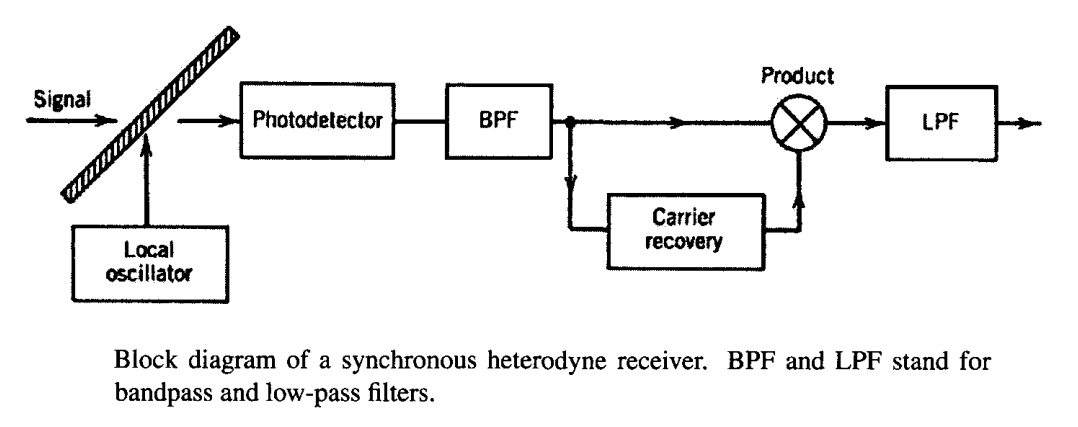The frequency of the local oscillator differs from the carrier frequency of the incident signal by the intermediate frequency (IF) chosen to be in the microwave region (~1 GHz). The current generated at the photodiode oscillates at the intermediate frequency and is passed through a bandpass filter (BPF) centered at this frequency ωIF. The filtered current, in the absence of noise, can be written aswhere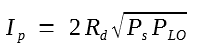and φ = φs - φLO is the phase difference between the signal and the local oscillator. The noise is also filtered by the BPF. Using the in-phase and out-of-phase quadrature components of the filtered Gaussian noise, the receiver noise is included through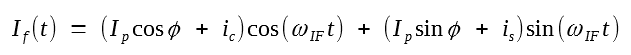where ic and is are Gaussian random variables of zero mean with variance σ2

In the case of synchronous demodulation, a clock circuit is used to recover the microwave carrier cos(ωIFt) as shown in the figure above. Then, If(t) is multiplied by this clock signal and filtered by a low-pass filter. The resulting baseband signal is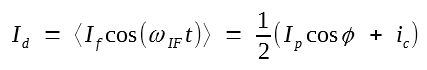where angle brackets denote low-pass filtering used for rejecting the ac components oscillating at 2ωIF. This equation shows that only the in-phase noise component affects the performance of a synchronous heterodyne receiver.

Synchronous demodulation requires recovery of the microwave carrier at the intermediate frequency ωIF. Several electronic schemes can be used for this purpose, all requiring a kind of electrical phase-locked loop. Two commonly used loops are the squaring loop and the Costas loop. A squaring loop uses a square-law device to obtain a signal of the form cos2IFt) that has a frequency component at 2ωIF. This component can be used to generate a microwave signal at ωIF.

A single-port receiver such as that shown in the figure above rejects half of the signal power Ps as well as half of the local-oscillator power PLO during the mixing process. The loss in signal power is equivalent to a 3-dB power penalty. A solution is provided by balanced receivers. As shown in the figure below schematically, a balanced heterodyne receiver employs a 3-dB coupler with two photodetectors at its two output ports. The operation of a balanced receiver can be understood by considering the photocurrents I+ and I- generated in each branch: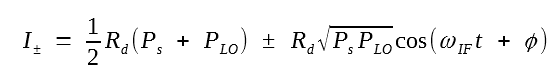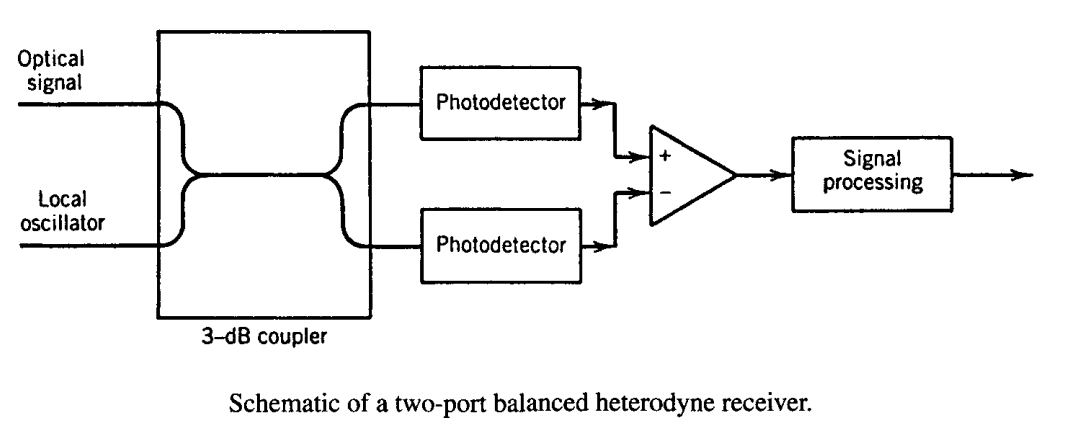The difference of the two currents, I+ - I-, provides the heterodyne signal. The dc term is eliminated completely during the subtraction process when the two branches are balanced in such a way that they recombine the signal and local-oscillator powers in a synchronized fashion. More importantly, such a balanced receiver uses all of the signal power and thus avoids the 3-dB power penalty intrinsic to any single-port receiver. At the same time, it helps to reduce the impact of intensity noise of a local oscillator, making it easier to operate the receiver in the shot-noise limit.

#### 2. Asynchronous Heterodyne Demodulation

The design of a heterodyne receiver can be simplified considerably by adopting the asynchronous demodulation scheme that does not require recovery of the microwave carrier. The figure below shows such a heterodyne receiver schematically.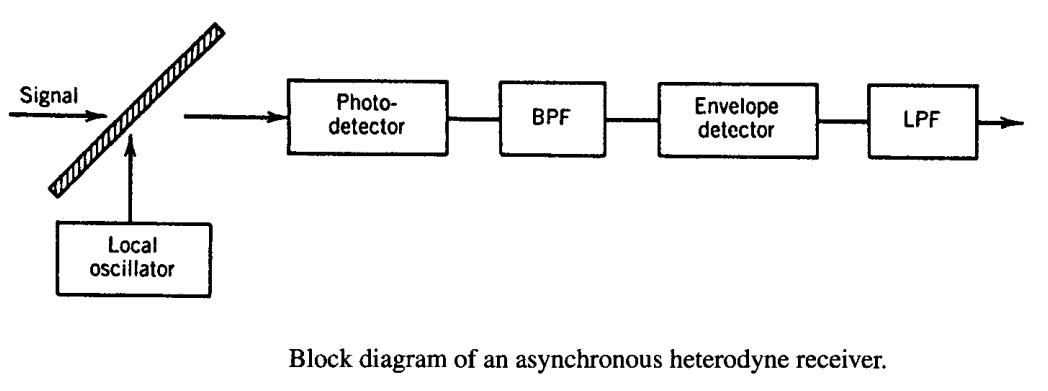As before, the current generated at the photodiode is passed through a BFP centered at the intermediate frequency ωIF. The filtered signal If(t) is converted to the baseband by an envelope detector, followed by a low-pass filter. The signal received by the decision circuit is just Id = |If|. It can be written as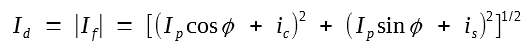The main difference is that both the in-phase and quadrature components of the receiver noise affect the signal. Although the SNR is somewhat degraded compared with the case of synchronous demodulation, sensitivity degradation resulting from the reduced SNR is relatively small (about 0.5 dB). As the phase-stability requirements are quite modest in the case of asynchronous demodulation, this scheme is often used for coherent lightwave systems. Similar to the case of synchronous receivers, it is common to employ a balanced receiver in the asynchronous case as well to avoid rejecting half of the signal and local-oscillator powers during the mixing process.

The asynchronous demodulation can be employed readily for both the amplitude shift keying (ASK) and frequency shift keying (FSK) formats. In the FSK case, the heterodyne receiver uses two separate branches to process the 1 and 0 bits whose carrier frequencies, and hence the intermediate frequencies, are different. The scheme can be used whenever the tone spacing is much larger than the bit rates, so that the spectra of 1 and 0 bits have negligible overlap. The two BPFs have their center frequencies separated exactly by the tone spacing so that each BPF passes either 1 or 0 bits only. A single-filter receiver of the above figure can be used for FSK demodulation if its bandwidth is chosen to be wide enough to pass the entire bit stream. The signal is then processed by a frequency discriminator to identify 1 and 0 bits. This scheme works well only if the tone spacing is less than or comparable to the bit rate.

Asynchronous demodulation cannot be used in the case of any PSK-based format because the phase of the transmitter laser and the local oscillator are not locked and can drift with time. The use of DPSK format permits asynchronous demodulation by using a delay scheme in which the filtered electrical signal is multiplied by a replica of it that has been delayed by one bit period. A better option is to implement the delay demodulation scheme in the optical domain because it bypasses the need of a local oscillator.

#### 3. Optical Delay Demodulation

In the case of differential phase encoding, a scheme known as delay demodulation can be employed. It makes use of a MZ interferometer whose two arms differ in lengths such that the signal in the longer arm is delayed by exactly one symbol duration (Ts = 1/Bs). Such a device is sometimes referred to as an optical delay interferometer. In contrast with a LiNbO3 MZ modulator that typically has only one output port, a delay interferometer, shown schematically in figure (a) below, is constructed by using two 3-dB couplers so that it has two output ports. When an optical field A(t) is incident on one of the input ports, the powers at the two output ports are given by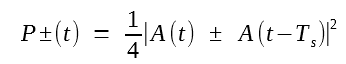where the choice of sign depends on whether the bar or the cross port of the MZ interferometer is used for photodetection. Such a demodulation scheme is also called self-coherent because a delayed replica of the optical signal itself is used in place of a local oscillator required for coherent detection.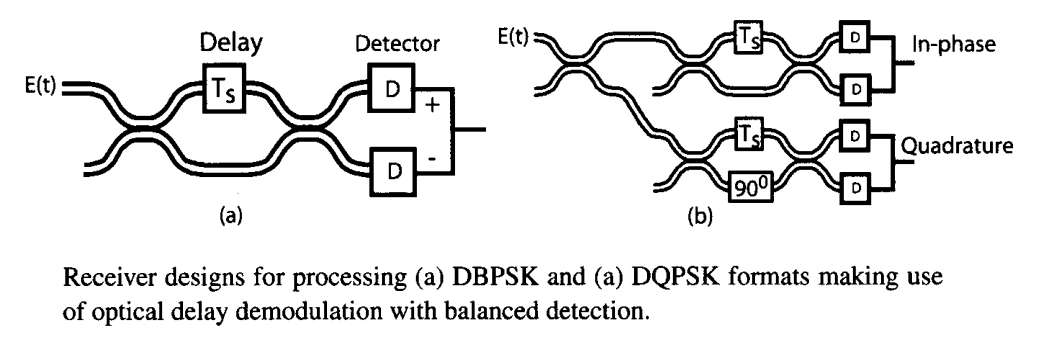Although phase information can be recovered by processing only one output of the MZ interferometer with a single photodetector, such a scheme is rarely used because it rejects half of the received power. In practice, receiver performance is improved considerably if two photodetectors are employed to detect both P±(t), and the resulting currents are subtracted. Such a balanced-detection scheme is shown schematically in figure (a) above.

Using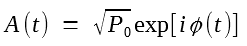with I± = RdP±(t), where Rd is the detector responsivity, the currents at the two photodetectors can be written as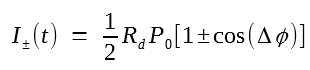where Δφ(t) = φ(t) - φ(t - Ts) is the phase difference between the two neighboring symbols. After subtracting the two currents, the signal used by the decision circuit is given by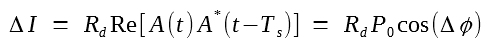In the BPSK case, Δφ = 0 or π depending on the bits transmitted. Thus, one can reconstruct the original bit stream from temporal variations of the electrical signal.

The situation is more complicated in the case of the DQPSK format. Figure (b) above shows the layout of a DQPSK receiver schematically. It employs two MZ interferometers with one-symbol delays but also introduces a relative phase shift of π/2 in one of them. The resulting two outputs of each MZ interferometer are then processed using the balanced detection scheme identical to that employed in the BPSK case. Because of the π/2 relative phase shift in one of the delay interferometers, two output currents correspond to the in-phase and quadrature components of the received optical field.

Optical delay interferometers can be fabricated with several technologies, including planar silica waveguides, LiNbO3 waveguides, and optical fibers. In all cases, it is important that the optical delay is precisely controlled because any deviation from the required delay Ts leads to degradation in the system performance. Since the optical path length of two arms may change because of environmental fluctuations, an active control of temperature is often required in practice. Free-space optics has also been used with success. The following figure shows a commercial DQPSK demodulator based on free-space optics. It employs an athermal design and is capable of operating at a symbol rate of 20 Gb/s in the entire C and L telecommunication bands. By 2010, commercial devices were capable of generating and receiving dual-polarization DPSK (or DQPSK) signals suitable for 100-Gb/s WDM channels.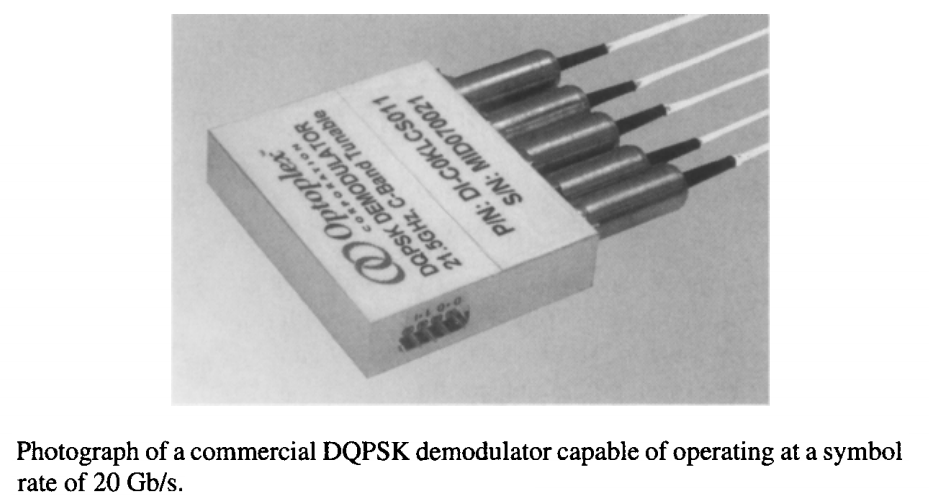An alternative design with reduced complexity makes use of a tunable birefringent element whose length is chosen such that the orthogonally polarized components are delayed with respect to each other exactly by one-symbol duration Ts. The following figure shows how such an element can be used to build a DQPSK receiver.When the input signal is polarized linearly at 45° with respect to the slow (or fast) axis of this element, and a polarization beam splitter (PBS) is used at its output ot split the orthogonally polarized components, the two outputs behave similar to the two outputs of an optical delay interferometer. In the case of the DBPSK format, these two outputs can be fed directly to a balanced detector. In the case of the DQPSK format, the device output is first split equally into two parts and phase shifts of ±45° are introduced using a polarization controller such that the two parts undergo a relative phase shift of π/2. The use of two balanced detectors then allows one to process the in-phase and quadrature components of the incident optical field separately. The main advantage of using a birefringent element is that an active control of temperature is not necessary because the two polarization components share the same optical path within this element. Moreover, its tuning capability allows one to operate such a receiver at different symbol rates.